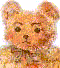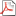BillHung.Net

Fuzzy Logic

http://www.seattlerobotics.org/encoder/mar98/fuz/fl_part2.html

FL (Fuzzy Logic) can control nonlinear systems that would be difficult or impossible to model mathematically. This opens doors for control systems that would normally be deemed unfeasible for automation.

http://www.mathworks.com/products/fuzzylogic/

# Fuzzy Logic Toolbox 2.2

## Design and simulate fuzzy logic systemsThe Fuzzy Logic Toolbox extends the MATLAB technical computing environment with tools for designing systems based on fuzzy logic. Graphical user interfaces (GUIs) guide you through the steps of fuzzy inference system design. Functions are provided for many common fuzzy logic methods, including fuzzy clustering and adaptive neurofuzzy learning.View data sheet (171k)